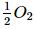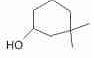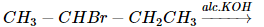Courses

# SRMJEEE Chemistry Mock Test - 6

## 35 Questions MCQ Test SRMJEEE Subject Wise & Full Length Mock Tests | SRMJEEE Chemistry Mock Test - 6

Description
This mock test of SRMJEEE Chemistry Mock Test - 6 for JEE helps you for every JEE entrance exam. This contains 35 Multiple Choice Questions for JEE SRMJEEE Chemistry Mock Test - 6 (mcq) to study with solutions a complete question bank. The solved questions answers in this SRMJEEE Chemistry Mock Test - 6 quiz give you a good mix of easy questions and tough questions. JEE students definitely take this SRMJEEE Chemistry Mock Test - 6 exercise for a better result in the exam. You can find other SRMJEEE Chemistry Mock Test - 6 extra questions, long questions & short questions for JEE on EduRev as well by searching above.
QUESTION: 1

Solution:
QUESTION: 2

Solution:
QUESTION: 3

### Ascorbic acid is a chemical name of

Solution:
QUESTION: 4

The IUPAC name of (C2H5)4C is

Solution:
QUESTION: 5

When H₂S gas is passed through the HCl containing aqueous solution of CuCl₂, HgCl₂, BiCl₃ and coCl₂, it does not precipitate out

Solution:
QUESTION: 6

Heaviest particle is

Solution:
QUESTION: 7

The greater the s- character in an orbital the ... is its energy

Solution:

The greater the s- character in an orbital, the shorter is the bond and greater is its strength. Thus, bond formed by sp hybridisation , as in acetylene is stronger as compare to ethane and ethene , because it has maximum bond energy.

QUESTION: 8

Electrophile in the case of chlorination of benzene in presence of FeCl₃ is

Solution:
QUESTION: 9

Sulphuric acid provides a simple example of

Solution:
QUESTION: 10

Which of the following is aromatic?

Solution:
QUESTION: 11

The acid showing salt like character in aqueous solutions is

Solution:
QUESTION: 12

60 J of heat flows out from 600 g of water at 30°C into surroundings at 25°C.The net entropy change in universe is approximately

Solution:
QUESTION: 13

A catalyst is used to

Solution:
QUESTION: 14

A glass bulb is filled with NO₂ gas and immersed in an ice bath at 0°C which becomes colourless after some time . This colourless gas will be

Solution:
QUESTION: 15

Calculate the heat of formation Δ H of CO (in kcal) from the following data :

C(graphite) + O2(g) → CO2 (g); ΔH = -94K. cal
CO(g) +(g) → CO2 (g) ; ΔH = -68K. cal

Solution:
QUESTION: 16

Aspirin is an acetylation product of

Solution:
QUESTION: 17

Which one of the following reactions occur at the cathode?

Solution:
QUESTION: 18

The IUPAC name ofis

Solution:
QUESTION: 19

The lightest metal is

Solution:

Lithium is lightest among all the metals with density 0.534 g/cm3.

QUESTION: 20

One mole of argon gas will have least density at

Solution:
QUESTION: 21

Which of the following applies to the reaction,1. CH₃CH = CHCH₃(major product)
2. CH₂ = CH − CH₂CH₃(minor product)

Solution:
QUESTION: 22

A compound having the formula NH₂CH₂COOH may behave

Solution:
QUESTION: 23

A symmetrical molecule has 2 chiral carbon atoms. The no. of isomers is

Solution: Explanation: For a molecule to be chiral, it must have a stereocenter and no axis of symmetry. An atom with a stereocenter has no identical bonds; it is a carbon atom with four unique substituents. There are two stereocenters in each of the three molecules.
QUESTION: 24

C-14 has a half-life of 5760 years. 100 mg of a sample containing C-14 is reduced to 25 mg in

Solution:
QUESTION: 25

Lacrymotor or tear gas is

Solution:
QUESTION: 26

Aniline reacts with concentrated HNO₃ to give :

Solution:
QUESTION: 27

Which is incorrect representation?

Solution:
QUESTION: 28

In Duma's method, the gas which is collected in Nitrometer is

Solution:
QUESTION: 29

Which of the following is used for making horses, shoe heels and stoppers?

Solution:
QUESTION: 30

The oxidation number of Pt in [Pt(C₂H₄)Cl₃]- is

Solution:
QUESTION: 31

Which of the following describes the hexagonal close packed arrangement of spheres?

Solution:
QUESTION: 32

If 100 ml of 1 N sulphuric acid were mixed with 100 ml of 1 M sodium hydroxide, the solution will be

Solution:
QUESTION: 33

The number of moles of H₂ in 0.224 L of hydrogen gas at STP (273 K, 1atm. assuming ideal gas behaviour is

Solution:
QUESTION: 34

The empirical formula of an organic compound containing carbon and hydrogen is CH2. The mass of one litre of this organic gas is exactly equal to that of one litre of nitrogen. Therefore the molecular formula of the organic gas is

Solution:
QUESTION: 35

When H₂S is passed through nitric acid, the product is

Solution: